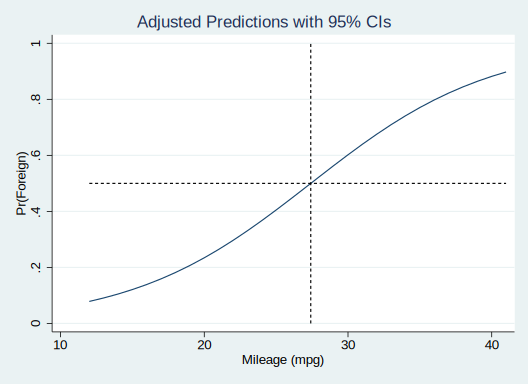In addition to the odds ratio interpretation of coefficients in a logistic regression model, the “divide by 4” rule can also help with interpretation.

. sysuse auto
(1978 Automobile Data)

. logit foreign mpg

Iteration 0:   log likelihood =  -45.03321
Iteration 1:   log likelihood = -39.380959
Iteration 2:   log likelihood = -39.288802
Iteration 3:   log likelihood =  -39.28864
Iteration 4:   log likelihood =  -39.28864

Logistic regression                             Number of obs     =         74
LR chi2(1)        =      11.49
Prob > chi2       =     0.0007
Log likelihood =  -39.28864                     Pseudo R2         =     0.1276

------------------------------------------------------------------------------
foreign |      Coef.   Std. Err.      z    P>|z|     [95% Conf. Interval]
-------------+----------------------------------------------------------------
mpg |   .1597621   .0525876     3.04   0.002     .0566922     .262832
_cons |  -4.378866   1.211295    -3.62   0.000    -6.752961   -2.004771
------------------------------------------------------------------------------



The coefficient on mpg, .1597, is the log odds. The “divide by 4” rule says that the log odds divided by 4 (so $$.1597/4 = .0399$$) is the maximum difference in predicted probability for a 1-unit change in mpg.

In other words, consider the following plot of predicted probabilities.

. quiet margins, at(mpg = (12(1)41)) nose

. marginsplot, recast(line) ///
>     addplot(pci .5 12 .5 41, lpattern(shortdash) lcolor(black)  || ///
>             pci 0 =4.378866/.1597621' 1 =4.378866/.1597621' , lpattern(shor
> tdash) lcolor(black)) ///
>             legend(off)

Variables that uniquely identify margins: mpgThe line represents the predicted probability of the outcome being 1 as mpg varies. In linear regression, this would be a straight line, and the difference in predicted probability between mpg = 14 versus mpg = 15 would be equivalent to the difference in predicted probability between mpg = 30 versus mpg = 31. However, since this is a logistic curve, the difference in predicted probability varies over mpg, being sharpest near the middle. Specifically, at the point when the predicted probability is exactly .5 (the dashed lines), the slope is the largest, and it recedes from there.

This means that at that middle point (around mpg = 27), the increasing mpg by 1 is increasing the predicted probability by .0399. For all other places on that curve, the increased probability is less than .0399.